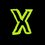# Inequalities: Solving Linear Inequalities

Hello ! This is the second note in the series Inequalities.



$\mathbf{LINEAR-INEQUALITIES}$

1. $a \geq b \Rightarrow$ either $a > b$ or $a = b$

2. If $a > b$ and $b > c \Rightarrow$ $a > c$ [ Transition property ]

3. For $a > b \Leftrightarrow a \pm c < b \pm c$

4. If $a > b$, then: \begin{aligned} ac > bc & \text{ if} & c > 0 \\ ac < bc & \text{ if} & c < 0 \end{aligned}

In other words, the sign of inequality flips if we multiply a negative real number to both side. For example: $3 > 2 \Leftrightarrow -3 < -2$ . Which is definitely true.

5. If $ax > b$, then: \begin{aligned} x > b/a & \text{ if} & a > 0 & \text{ [Sign remains unchanged] }\\ x < b/a & \text{ if} & a < 0 & \text{ [Sign changes] } \end{aligned}

In other words, the sign of inequality flips if we divide both sides by a negative real number.



$\mathbf{SOLVED-EXAMPLE}$ 1. Find the range of values of $x$ satisfying the inequality: $3 \geq 1-2x > -19$ Solution: \begin{aligned} -3 \leq 2x-1& < 19 & \text{ [Multiplying both sides by -1] } \\ -2 \leq 2x & < 20 & \\ -1 \leq x & < 10 & \text{ [Dividing both sides by 2] } \end{aligned} Thus the answer is: $x \in [-1,10)$



$\mathbf{PRACTICE}$ 1. Find the range of values of $y$ in interval form, satisfying the inequality: $-4 \leq \dfrac{y-1}{3} \leq a$ Where $'a'$ is number of irrational roots of: $\sqrt{x^2 + \sqrt{x^2 + 11}} + \sqrt{x^2 - \sqrt{x^2 + 11}} = 4$



3. Frame your own questions and feel free to share it down below in the comments.

I thank each one of you for reading this note. Comment below your views , answers and share interesting questions related to it.

Stay tuned for more notes and questions. You can even post your matter down here in the comments to make this topic more interesting. (I've reserved the advanced topics and properties for upcoming notes.)

Use #PSQuest to see more from me.

Complete set here : Inequalities

Like and Share :)Note by Priyansh Sangule
7 years ago

This discussion board is a place to discuss our Daily Challenges and the math and science related to those challenges. Explanations are more than just a solution — they should explain the steps and thinking strategies that you used to obtain the solution. Comments should further the discussion of math and science.

When posting on Brilliant:

• Use the emojis to react to an explanation, whether you're congratulating a job well done , or just really confused .
• Ask specific questions about the challenge or the steps in somebody's explanation. Well-posed questions can add a lot to the discussion, but posting "I don't understand!" doesn't help anyone.
• Try to contribute something new to the discussion, whether it is an extension, generalization or other idea related to the challenge.

MarkdownAppears as
*italics* or _italics_ italics
**bold** or __bold__ bold
- bulleted- list
• bulleted
• list
1. numbered2. list
1. numbered
2. list
Note: you must add a full line of space before and after lists for them to show up correctly
paragraph 1paragraph 2

paragraph 1

paragraph 2

[example link](https://brilliant.org)example link
> This is a quote
This is a quote
    # I indented these lines
# 4 spaces, and now they show
# up as a code block.

print "hello world"
# I indented these lines
# 4 spaces, and now they show
# up as a code block.

print "hello world"
MathAppears as
Remember to wrap math in $$ ... $$ or $ ... $ to ensure proper formatting.
2 \times 3 $2 \times 3$
2^{34} $2^{34}$
a_{i-1} $a_{i-1}$
\frac{2}{3} $\frac{2}{3}$
\sqrt{2} $\sqrt{2}$
\sum_{i=1}^3 $\sum_{i=1}^3$
\sin \theta $\sin \theta$
\boxed{123} $\boxed{123}$